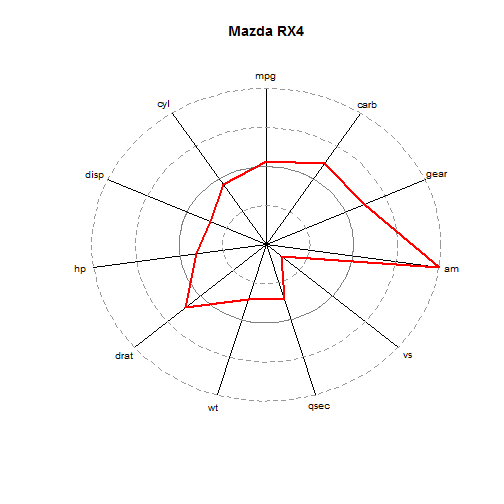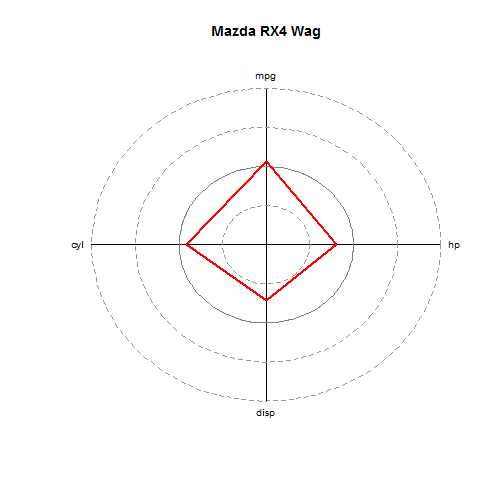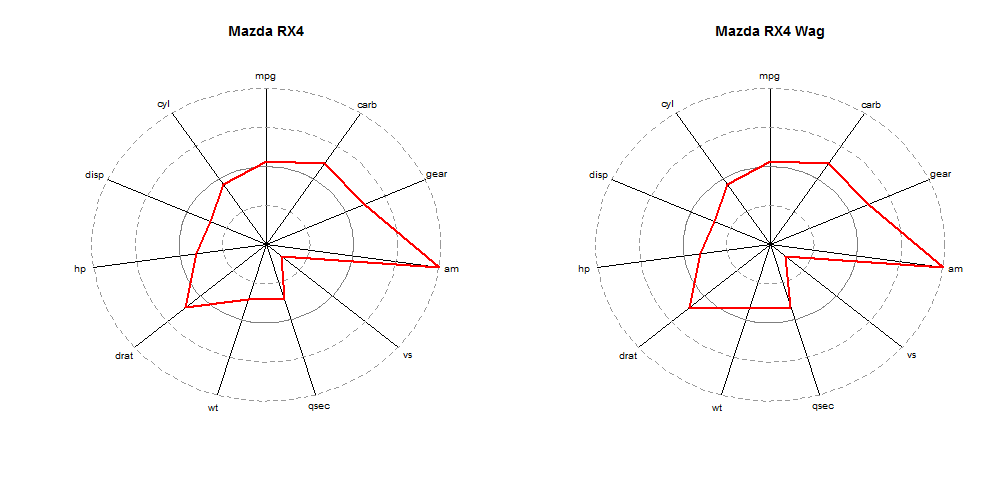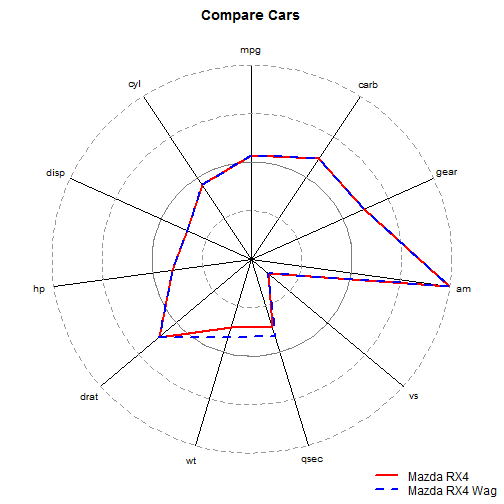## Tuesday, November 5, 2013

### Spider Web Plots in R

**Edit** I found a similar plotting technique using radarchart from the fmsb package, found at http://artax.karlin.mff.cuni.cz/r-help/library/fmsb/html/radarchart.html

Unless your brain works differently than mine, then polar coordinates aren't great for being precise in consuming and measuring data. Use bar charts, not pie charts, right angles! That being said, “spider plots” or “web plots” or whatever you want to call these charts, can succinctly summarize a lot of information, and are visually pleasing.

Here's a function to create this kind of plot.

``````
webplot = function(data, data.row = NULL, y.cols = NULL, main = NULL, add = F,
col = "red", lty = 1, scale = T) {
if (!is.matrix(data) & !is.data.frame(data))
stop("Requires matrix or data.frame")
if (is.null(y.cols))
y.cols = colnames(data)[sapply(data, is.numeric)]
if (sum(!sapply(data[, y.cols], is.numeric)) > 0) {
out = paste0("\"", colnames(data)[!sapply(data, is.numeric)], "\"",
collapse = ", ")
stop(paste0("All y.cols must be numeric\n", out, " are not numeric"))
}
if (is.null(data.row))
data.row = 1
if (is.character(data.row))
if (data.row %in% rownames(data)) {
data.row = which(rownames(data) == data.row)
} else {
stop("Invalid value for data.row:\nMust be a valid rownames(data) or row-index value")
}
if (is.null(main))
main = rownames(data)[data.row]
if (scale == T) {
data = scale(data[, y.cols])
data = apply(data, 2, function(x) x/max(abs(x)))
}
data = as.data.frame(data)
n.y = length(y.cols)
polar.vals = (90 + seq(0, 360, length.out = n.y + 1)) * pi/180

#
plot(0, xlim = c(-2.2, 2.2), ylim = c(-2.2, 2.2), type = "n", axes = F,
xlab = "", ylab = "")
title(main)
lapply(polar.vals, function(x) lines(c(0, 2 * cos(x)), c(0, 2 * sin(x))))
lapply(1:n.y, function(x) text(2.15 * cos(polar.vals[x]), 2.15 * sin(polar.vals[x]),
y.cols[x], cex = 0.8))

lapply(seq(0.5, 2, 0.5), function(x) lines(x * cos(seq(0, 2 * pi, length.out = 100)),
x * sin(seq(0, 2 * pi, length.out = 100)), lwd = 0.5, lty = 2, col = "gray60"))
lines(cos(seq(0, 2 * pi, length.out = 100)), sin(seq(0, 2 * pi, length.out = 100)),
lwd = 1.2, col = "gray50")
}

r = 1 + data[data.row, y.cols]
xs = r * cos(polar.vals)
ys = r * sin(polar.vals)
xs = c(xs, xs)
ys = c(ys, ys)

lines(xs, ys, col = col, lwd = 2, lty = lty)

}
``````

Using the mtcars data set:

``````webplot(mtcars)
``````Basically, we take a data frame, scale all numeric columns, and then plot how far above/below average (the thick black line) each of the values are for a particular row of data.

Here are the Arguments:

• data - data.frame or matrix
• data.row - row of data to plot (if NULL uses row 1)
• y.cols - columns of interest (if NULL it selects all numeric columns)
• main - title of plot (if NULL then rowname of data)
• add - whether the plot should be added to an existing plot
• col - color of the data line
• lty - lty of the data line

For example, we can change the data row that we plot, as well as the columns we want to include

``````webplot(mtcars, 2, y.cols = c("mpg", "cyl", "disp", "hp"))
``````It turns out that this isn't the easiest way to compare results, it might be helpful to overlay plots

``````par(mfcol = c(1, 2))
webplot(mtcars, "Mazda RX4")
webplot(mtcars, "Mazda RX4 Wag")
````````````par(mfcol = c(1, 1))
``````
``````par(mar = c(1, 1, 2, 1))
webplot(mtcars, "Mazda RX4", main = "Compare Cars")
webplot(mtcars, "Mazda RX4 Wag", add = T, col = "blue", lty = 2)
par(new = T)
par(mar = c(0, 0, 0, 0))
plot(0, type = "n", axes = F)
legend("bottomright", lty = c(1, 2), lwd = 2, col = c("red", "blue"), c("Mazda RX4",
"Mazda RX4 Wag"), bty = "n")
``````Which makes it easy to observe that the Mazda RX4 and Mazda RX4 Wagon are very similar, with the Wagon being a little heavier, and takes a little longer to travel a quarter mile

1.Great effort. Thanks a lot. A way to draw polygons, i.e. fill the areas would be nice. Your functions works also very well interactively... I created a shiny based demo: http://glimmer.rstudio.com/mbannert/radar_chart/
will also look to implement comparisons at some point.

1.Very cool Shiny App, 114385797025766597348!

2.Not timely, I know, but this adds a fill option with transparency

webplot = function(data, data.row = NULL, y.cols = NULL, main = NULL, add = F,
col = "red", lty = 1, scale = T, fill=F, alpha=.6) {
if (!is.matrix(data) & !is.data.frame(data))
stop("Requires matrix or data.frame")
if (is.null(y.cols))
y.cols = colnames(data)[sapply(data, is.numeric)]
if (sum(!sapply(data[, y.cols], is.numeric)) > 0) {
out = paste0("\"", colnames(data)[!sapply(data, is.numeric)], "\"",
collapse = ", ")
stop(paste0("All y.cols must be numeric\n", out, " are not numeric"))
}
if (is.null(data.row))
data.row = 1
if (is.character(data.row))
if (data.row %in% rownames(data)) {
data.row = which(rownames(data) == data.row)
} else {
stop("Invalid value for data.row:\nMust be a valid rownames(data) or row-index value")
}
if (is.null(main))
main = rownames(data)[data.row]
if (scale == T) {
data = scale(data[, y.cols])
data = apply(data, 2, function(x) x/max(abs(x)))
}
data = as.data.frame(data)
n.y = length(y.cols)
polar.vals = (90 + seq(0, 360, length.out = n.y + 1)) * pi/180

plot(0, xlim = c(-2.2, 2.2), ylim = c(-2.2, 2.2), type = "n", axes = F,
xlab = "", ylab = "")
title(main)
lapply(polar.vals, function(x) lines(c(0, 2 * cos(x)), c(0, 2 * sin(x))))
lapply(1:n.y, function(x) text(2.15 * cos(polar.vals[x]), 2.15 * sin(polar.vals[x]),
y.cols[x], cex = 0.8))

lapply(seq(0.5, 2, 0.5), function(x) lines(x * cos(seq(0, 2 * pi, length.out = 100)),
x * sin(seq(0, 2 * pi, length.out = 100)), lwd = 0.5, lty = 2, col = "gray60"))
lines(cos(seq(0, 2 * pi, length.out = 100)), sin(seq(0, 2 * pi, length.out = 100)),
lwd = 1.2, col = "gray50")
}

newcol_temp = c(col2rgb(col))/255
newcol = rgb(newcol_temp,newcol_temp,newcol_temp,alpha)

r = 1 + data[data.row, y.cols]
xs = r * cos(polar.vals)
ys = r * sin(polar.vals)
xs = c(xs, xs)
ys = c(ys, ys)

if(fill==T) {
polygon(xs, ys, col = newcol, lwd = 2, lty = lty, border=F)
} else if(fill==F) {
lines(xs, ys, col = newcol, lwd = 2, lty = lty)
}

}

2.thank you. awesome function you got there.
btw how to add scale tickmark in each dataframe component (disp, cyl, mpg etc) in the plot?

1.I'm not 100% sure what you are asking for - are you asking about something that is currently in the plot, or are you asking how you would add something -to- the chart

3.oh, and 1 more questions. i like command add = T and want to add multiple webplot for each row (for example row 2-100) in my data. so far, i copy and paste command webplot (mtcars, 2:100) manually. is there a loop command to accomodate this? thank you very much. great blog!

1.I haven't thought about how to do that efficiently - I think I would strip apart the function and simply write a loop around the "if(add==T..." portion. My take was that it is rather difficult to do more than pairwise comparisons, but that might be worth looking into

4.Please I have median data for drugs A, B, C, D and E against a particular organism as follows: 40%, 53%, 67%, 72% and 82%. Please how do I input this data to be able to plot a spider chart